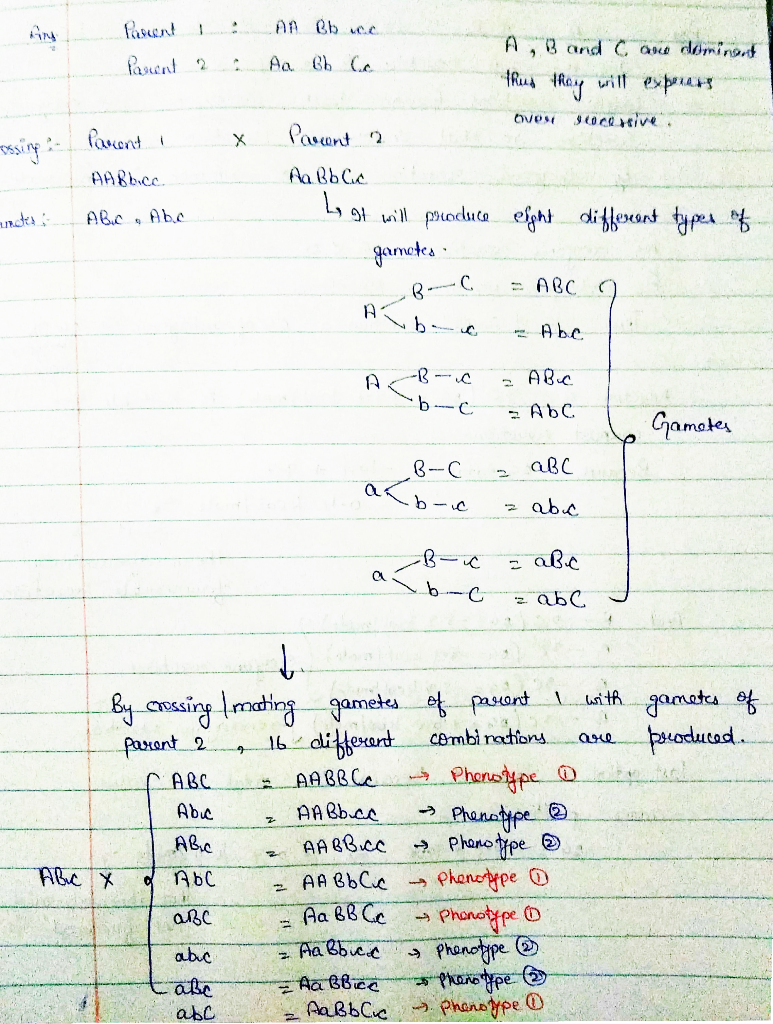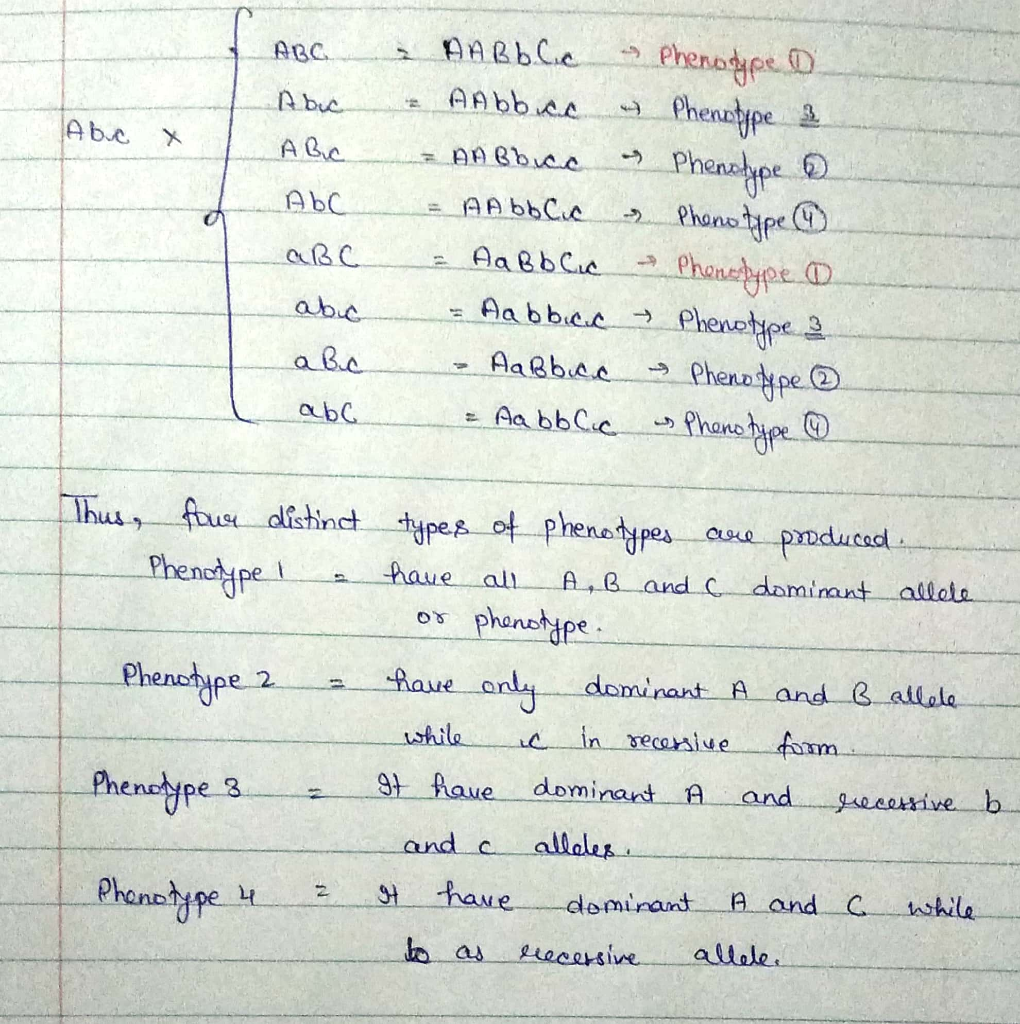In: Biology

# Parent 1: AA Bb cc Parent 2: Aa Bb Cc A, B, and C are dominant....

Parent 1: AA Bb cc

Parent 2: Aa Bb Cc

A, B, and C are dominant.

How many distinct phenotypes could be produced?

## Solutions

##### Expert Solution## Related Solutions

##### 5. Consider the following tetrahybrid self-cross: Aa Bb Cc Dd    X    Aa Bb Cc Dd Calculate...
5. Consider the following tetrahybrid self-cross: Aa Bb Cc Dd    X    Aa Bb Cc Dd Calculate the probability for each of the following offspring. Show your work. Genotype Aa BB CC Dd Heterozygous for ALL FOUR genes Heterozygous for ANY gene Dominant phenotype for ALL FOUR traits GIVEN that an individual offspring has the dominant phenotype for all four traits, what is the probability that individual is heterozygous for all four genes? GIVEN that an individual offspring has the recessive...
##### An organism is heterozygous at three genetic loci (Aa, Bb and Cc) located on different chromosomes....
An organism is heterozygous at three genetic loci (Aa, Bb and Cc) located on different chromosomes. Explain how these alleles are transmitted by the process of A) mitosis to daughter cells, and B) meiosis to gametes Show the final genetic composition of the daughter cells or gametes with respect to these loci? C. How many different allelic combinations are possible with 8 heterozygous loci on 8 different chromosomes?
##### Peas heterozygous for three independently assorting genes were intercrossed. (example cross: Aa Bb Cc x Aa...
Peas heterozygous for three independently assorting genes were intercrossed. (example cross: Aa Bb Cc x Aa Bb Cc ) What proportion of the offspring will be homozygous for one gene and heterozygous for the other two? (hint: apply binomial expansion equation)    1/4     3/8     1/8     3/16     1/2 already answered 1/4 and 1/2 and they were incorrect
##### A man (Bob) is heterozygous for Aa Bb Cc, where the genes show independent assortment: He...
A man (Bob) is heterozygous for Aa Bb Cc, where the genes show independent assortment: He does not have a known phenotype, and neither does their mother; but their father does. (A third eye). We know it has to be associated with one of the three given loci. He has 3 three children (1 boy and 2 girls) with a woman who is also phenotypically normal, of which one girl has a third eye. Based on this information, what type...
##### A fair coin is tossed three times and the events AA, BB, and CC are defined as follows: A:{A:{ At least...
A fair coin is tossed three times and the events AA, BB, and CC are defined as follows: A:{A:{ At least one head is observed }}  B:{B:{ At least two heads are observed }}  C:{C:{ The number of heads observed is odd }} Find the following probabilities by summing the probabilities of the appropriate sample points (note that 0 is an even number): (a)  P(not C)P(not C) ==   (b)  P((not A) and B)P((not A) and B) ==   (c)  P((not A) or B or C)P((not A) or B or C) ==
##### 3. Morris Industries manufactures and sells three products (AA, BB, and CC). The sales price and...
3. Morris Industries manufactures and sells three products (AA, BB, and CC). The sales price and unit variable cost for the three products are as follows: Product Sales Price per Unit Variable Cost per Unit AA $55$25      BB 45      20      CC 30      5      Their sales mix is reflected as a ratio of 5:3:2. Annual fixed costs shared by the three products are \$275,000 per year. A. What are total variable costs for Morris with their current product mix? Total...
##### 1. Consider the regular grammar G given below: S → aS|aA|bB|λ A → aA|bS B →...
1. Consider the regular grammar G given below: S → aS|aA|bB|λ A → aA|bS B → bB|aS (a) Give a derivation for the strings aabbba and baaba (b) Give an infinite language L such that L is not a subset of L(G) (c) Give a regular expression that describes the language L(G).
##### Assuming independent assortment of each gene, in the cross female mouse: Aa Bb cc Dd ee...
Assuming independent assortment of each gene, in the cross female mouse: Aa Bb cc Dd ee FF x male mouse: Aa bb Cc Dd ee fY. Assume all of these genes have simple dominance/recessive relationships. The F/f genetic locus is on the X chromosome in mice. (a). What is the expected ratio of F1 progeny that will be phenotypically identical to the female parent? (b) What ratio of progeny will be genotypically identical to the female? (c) . What ratio...
##### If the a and b loci are 20 cM apart and an AA BB individual and...
If the a and b loci are 20 cM apart and an AA BB individual and an aa bb individual mate: What gametes will the F1 individuals produce, and in what proportions? What phenotypic classes in what proportions are expected in the F2 generation (assuming complete dominance for both genes) (show procedure, NO score for direct result)?
##### If the a and b loci are 20 cM apart and an AA BB individual and...
If the a and b loci are 20 cM apart and an AA BB individual and an aa bb individual mate: What gametes will the F1 individuals produce, and in what proportions? What phenotypic classes in what proportions are expected in the F2 generation (assuming complete dominance for both genes) (show procedure)